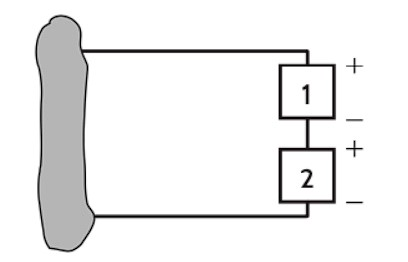Home / Expert Answers / Electrical Engineering / q5-nbsp-in-nbsp-fig-p1-24-watts-how-much-power-is-absorbed-by-element-2-element-1-9-volts-pa334

# (Solved): (Q5) In Fig, P1 = 24 Watts. How much power is absorbed by element 2 ? (Element 1 = 9 Volts ...

(Q5) In Fig, P1 = 24 Watts. How much power is absorbed by element 2 ?

(Element 1 = 9 Volts, Element 2 = 5 Volts)Notes on entering solution:

Enter your solution to the nearest hundredth of a Watt

ex. 5W is entered as 5.00